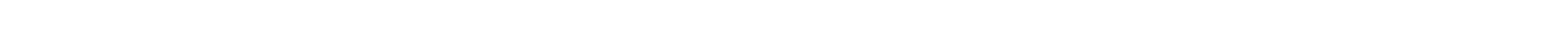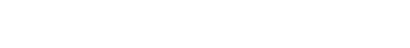## C# Tutorial

Nullable are special data types that can be assigned normal data values as well a null values. For example: Nullable<Int32> can be assigned any integer value from -2,147,483,648 to 2,147,483,647 as well as null. Similarly Nullable<bool> can be assigned true, false and null.The syntax for declaring a nullable in C# is given as follows:DataType? VarName = value;In the above syntax, DataType is the data type such as it, float etc. followed by ?. VarName is the name of the variable. The variable is assigned a value which can be null or any value of the specified data type.A program that demonstrates nullables in C# is given as follows:Source Code: Program that demonstrates nullables in C#using System; namespace NullablesDemo {   class Example   {      static void Main(string[] args)      {         int? val1 = 4;         int? val2 = null;         bool? val3 = true;         bool? val4 = null;         char? val5 = 'a';         char? val6 = null;         Console.WriteLine("Value of val1: {0}", val1);         Console.WriteLine("Value of val2: {0}", val2);         Console.WriteLine("Value of val3: {0}", val3);         Console.WriteLine("Value of val4: {0}", val4);         Console.WriteLine("Value of val5: {0}", val5);         Console.WriteLine("Value of val6: {0}", val6);      }   } }The output of the above program is as follows:Value of val1: 4 Value of val2: Value of val3: True Value of val4: Value of val5: a Value of val6:Now let us understand the above program.The int nullable types val1 and val2 store 4 and null respectively. The bool nullable types val3 and val4 store true and null respectively. The char nullable types val5 and val6 store a and null respectively. The code snippet for this is as follows:int? val1 = 4;         int? val2 = null;         bool? val3 = true;         bool? val4 = null;         char? val5 = 'a';         char? val6 = null;After that, the values of all the data variables are displayed. The code snippet for this is as follows: Console.WriteLine("Value of val1: {0}", val1);         Console.WriteLine("Value of val2: {0}", val2);         Console.WriteLine("Value of val3: {0}", val3);         Console.WriteLine("Value of val4: {0}", val4);         Console.WriteLine("Value of val5: {0}", val5);         Console.WriteLine("Value of val6: {0}", val6);Null Coalescing OperatorThe null coalescing operator is represented as ??. It has two operands and the operator returns the value of the first operand if it is not null. However if the first operand is null, then the operator returns the value of the second operand.A program that demonstrates the null coalescing operator in C# is given as follows:Source Code: Program that demonstrates null coalescing operator in C#using System; namespace NullCoalescingOperatorDemo {   class Example   {      static void Main(string[] args)      {         int? num1 = 5;         int? num2 = null;         int? num3 = 2;         Console.WriteLine(" num1 ?? num2: {0}", num1 ?? num2);         Console.WriteLine(" num2 ?? num3: {0}", num2 ?? num3);      }   } }The output of the above program is as follows:num1 ?? num2: 5 num2 ?? num3: 2Now let us understand the above program.The int nullable types num1, num2 and num3 store 5, null and 2 respectively. When the null coalescing operator is used on num1 and num2, 5 is printed as the first operand i.e. num1 is not null. When the null coalescing operator is used on num2 and num3, 2 is printed as the first operand i.e. num2 is null. The code snippet for this is given as follows:  int? num1 = 5;         int? num2 = null;         int? num3 = 2;         Console.WriteLine(" num1 ?? num2: {0}", num1 ?? num2);         Console.WriteLine(" num2 ?? num3: {0}", num2 ?? num3);

# Nullables in C#

Nullable are special data types that can be assigned normal data values as well a null values. For example: Nullable<Int32> can be assigned any integer value from -2,147,483,648 to 2,147,483,647 as well as null. Similarly Nullable<bool> can be assigned true, false and null.

The syntax for declaring a nullable in C# is given as follows:

DataType? VarName = value;

In the above syntax, DataType is the data type such as it, float etc. followed by ?. VarName is the name of the variable. The variable is assigned a value which can be null or any value of the specified data type.

A program that demonstrates nullables in C# is given as follows:

Source Code: Program that demonstrates nullables in C#

using System;
namespace NullablesDemo
{
class Example
{
static void Main(string[] args)
{
int? val1 = 4;
int? val2 = null;
bool? val3 = true;
bool? val4 = null;
char? val5 = 'a';
char? val6 = null;
Console.WriteLine("Value of val1: {0}", val1);
Console.WriteLine("Value of val2: {0}", val2);
Console.WriteLine("Value of val3: {0}", val3);
Console.WriteLine("Value of val4: {0}", val4);
Console.WriteLine("Value of val5: {0}", val5);
Console.WriteLine("Value of val6: {0}", val6);
}
}
}

The output of the above program is as follows:

Value of val1: 4
Value of val2:

Value of val3: True
Value of val4:

Value of val5: a
Value of val6:

Now let us understand the above program.

The int nullable types val1 and val2 store 4 and null respectively. The bool nullable types val3 and val4 store true and null respectively. The char nullable types val5 and val6 store a and null respectively. The code snippet for this is as follows:

int? val1 = 4;
int? val2 = null;
bool? val3 = true;
bool? val4 = null;
char? val5 = 'a';
char? val6 = null;

After that, the values of all the data variables are displayed. The code snippet for this is as follows:

 Console.WriteLine("Value of val1: {0}", val1);
Console.WriteLine("Value of val2: {0}", val2);
Console.WriteLine("Value of val3: {0}", val3);
Console.WriteLine("Value of val4: {0}", val4);
Console.WriteLine("Value of val5: {0}", val5);
Console.WriteLine("Value of val6: {0}", val6);

## Null Coalescing Operator

The null coalescing operator is represented as ??. It has two operands and the operator returns the value of the first operand if it is not null. However if the first operand is null, then the operator returns the value of the second operand.

A program that demonstrates the null coalescing operator in C# is given as follows:

Source Code: Program that demonstrates null coalescing operator in C#

using System;
namespace NullCoalescingOperatorDemo
{
class Example
{
static void Main(string[] args)
{
int? num1 = 5;
int? num2 = null;
int? num3 = 2;
Console.WriteLine(" num1 ?? num2: {0}", num1 ?? num2);
Console.WriteLine(" num2 ?? num3: {0}", num2 ?? num3);
}
}
}

The output of the above program is as follows:

num1 ?? num2: 5
num2 ?? num3: 2

Now let us understand the above program.

The int nullable types num1, num2 and num3 store 5, null and 2 respectively. When the null coalescing operator is used on num1 and num2, 5 is printed as the first operand i.e. num1 is not null. When the null coalescing operator is used on num2 and num3, 2 is printed as the first operand i.e. num2 is null. The code snippet for this is given as follows:

  int? num1 = 5;
int? num2 = null;
int? num3 = 2;
Console.WriteLine(" num1 ?? num2: {0}", num1 ?? num2);
Console.WriteLine(" num2 ?? num3: {0}", num2 ?? num3);#### austin faith

Avery good write-up. Please let me know what are the types of C# libraries used for AI development.#### kariya arti

very satisfied!!#### jean-Francois Michaud

Good tutorial. Small question: Say, there is : enum numbers { one, two, three} and a string field_enum ="one" how would I from the variable field_enum have a response with value numbers.one so that it can be treated as an enum and not as a string. making a list from the enum, and loop into the list. is not elegant... and may not work is forced value on field is forced ( one = 100).#### Kshitiz

Hi Team Knowledge Hut, Thank you for such an informative post like this. I am completely new to this digital marketing field and do not have much idea about this, but your post has become a supportive pillar for me. After reading the blog I would expect to read more about the topic. I wish to get connected with you always to have updates on these sorts of ideas. Regards, Kshitiz#### Ed

The reason abstraction can be used with this example is because, the triangle, circle. Square etc can be defined as a shape, for example.....shape c = new circle(5,0)...the abstract object c now points at the circle class. Thus hiding implementation

## Swift Tutorial

Introduction to Swift Tutorial

R Programming

Python Tutorial